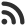Feed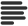Articles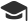Tutorials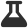CodingLab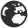CommunityLeaderboardFounder & Programmer at HackersFriend Updated Nov. 19, 2019, 12:23 a.m. ⋅ 99 views

# Efficient Method to Check if a Number is Multiple of 3

We are given a number and we want to check if the number is Multiple of 3 or not. The very simple first solution comes in our mind is old school way. We can check if a number is multiple of 3 or not by adding all the digits of number. If the total sum of digits is multiple of 3 then the number is also multiple of 3 otherwise it is not.

This is simplest way of doing it. We can be more efficient at doing this.

Efficient approach

They idea here is to look into the binary repersentation of the given number. In binary representation of the number, If difference between count of odd set bits (Bitsets at odd positions) and even set bits is multiple of 3 then  the number is also multiple of 3.

Algorithm

``````isMutlipleOf3(n):

Step 1: Make n positive if n is negative.

Step 2: If number is 0 then return 1

Step 3: If number is 1 then return 0

Step 4: Initialize: oddsCount = 0, evenCount = 0

Step 5: Loop while n != 0
{
Increment oddCount if rightmost bit is set

Right-shift n by 1 bit

Increment evenCount If rightmost bit is set

Right-shift n by 1 bit
}

return isMutlipleOf3(oddCount - evenCount)``````

Time complexity of this approach would be O(log n)

Implementation

Let's code the above algorithm.

``````// C++ program to check if n is a multiple of 3 efficiently

#include <bits/stdc++.h>
using namespace std;

int isMultipleOf3(int n)
{
int oddCount = 0, evenCount = 0;

// Make no positive. We are doing this to avoid stack overflow in recursion

if (n < 0)
n = -n;
if (n == 0)
return 1;
if (n == 1)
return 0;

while (n) {
/* If odd bit is set then
increment odd counter */

if (n & 1)
oddCount++;

/* If even bit is set then
increment even counter */
if (n & 2)
evenCount++;
n = n >> 2;
}

return isMultipleOf3(abs(oddCount - evenCount));
}

// Main function

int main()
{
int n = 33;
if (isMultipleOf3(n))

printf("%d is multiple of 3", num);
else

printf("%d is not a multiple of 3", num);
return 0;
}
``````

 arrow_upward UpvoteComment arrow_downward downvote

Events

Nov. 28, 2018, 5:30 p.m.

Python from zero to hero

place Delhi

Aug. 13, 2018, 5:30 p.m.

Python from zero to hero

place Bangalore ( HackersFriend office BTM Layout)